Next: Experimental Results Up: Computer Vision Based Method Previous: Spatial Wavelet Analysis

# Decision Fusion

In this section, we describe a voting based decision fusion strategy. However, other data fusion methods can be also used to combine the decision of four stages of the flame and fire detection algorithm.

Voting schemes include unanimity voting, majority voting, and m-out-of-n voting in which an output choice is accepted if at least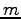votes agree with the decisions of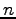sensors . We use a variant of-out-of-voting, the so-called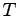-out-of-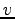voting in which the output is accepted if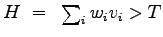where the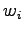's are user-defined weights, the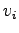's are decisions of the four stages of the algorithm, andis a user-defined threshold. Decision parametercan take binary values 0 and 1, corresponding to normal case and the existence of fire, respectively. The decision parameter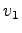is 1 if the pixel is a moving pixel, and 0 if it is stationary. The decision parameter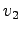is taken as 1 if the pixel is fire-colored, and 0 otherwise. The decision parameter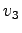is 1 if the number of zero crossings of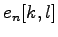and/or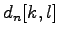in a few seconds exceed a threshold value, and 0 otherwise. The decision parameter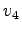is defined in Equation ().

In uncontrolled fire, it is expected that the fire region should have a non-convex boundary. To gain a further robustness to false alarms, another step checking the convexity of the fire region is also added to the proposed algorithm. Convexity of regions is verified in a heuristic manner. Boundaries of the regions in the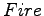mask are checked for their convexity along equally spaced five vertical and five horizontal lines using a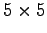grid. The analysis simply consists of checking whether the pixels on each line belong to the region or not. If at least three consecutive pixels belong to the background, then this region violates the convexity condition. Amask region which has background pixels on the intersecting vertical and/or horizontal lines, is assumed to have a non-convex boundary. This eliminates false alarms due to match light sources, sun, etc.Next: Experimental Results Up: Computer Vision Based Method Previous: Spatial Wavelet Analysis
ugur toreyin 2005-11-27